## Maxwell’s Equations

Maxwell’s equations help form the foundation of classical electrodynamics, optics, and electric circuits.

### Learning Objectives

Explain the meaning and importance of Maxwell’s equations

### Key Takeaways

#### Key Points

• Maxwell’s four equations describe how electric charges and currents create electric and magnetic fields, and how they affect each other.
• Gauss’s law relates an electric field to the charge(s) that create(s) it.
• Gauss’s law for magnetism states that there are no “magnetic charges” analogous to electric charges, and that magnetic fields are instead generated by magnetic dipoles.
• Faraday’s law describes how a time-varying magnetic field (or flux ) induces an electric field. The principle behind this phenomenon is used in many electric generators.
• Ampere ‘s law originally stated that a magnetic field is created by an electrical current. Maxwell added that a changing electric flux can also generate a magnetic field.

#### Key Terms

• differential equation: An equation involving the derivatives of a function.
• flux: A quantitative description of the transfer of a given vector quantity through a surface. In this context, we refer to the electric flux and magnetic flux.

### Maxwell’s Equations

Maxwell’s equations are a set of four partial differential equations that, along with the Lorentz force law, form the foundation of classical electrodynamics, classical optics, and electric circuits.

Named after esteemed physicist James Clerk Maxwell, the equations describe the creation and propagation of electric and magnetic fields. Fundamentally, they describe how electric charges and currents create electric and magnetic fields, and how they affect each other.

Maxwell’s equations can be divided into two major subsets. The first two, Gauss’s law and Gauss’s law for magnetism, describe how fields emanate from charges and magnets respectively. The other two, Faraday’s law and Ampere’s law with Maxwell’s correction, describe how induced electric and magnetic fields circulate around their respective sources.

Each of Maxwell’s equations can be looked at from the “microscopic” perspective, which deals with total charge and total current, and the “macroscopic” set, which defines two new auxiliary fields that allow one to perform calculations without knowing microscopic data like atomic-level charges.

### Gauss’s Law

Gauss’s law relates an electric field to the charge(s) that create(s) it. The field (E) points towards negative charges and away from positive charges, and from the microscopic perspective, is related to charge density (ρ) and vaccuum permittivity (ε0, or permittivity of free space) as:

$\nabla \cdot \bf \text{E}=\frac {\rho}{\epsilon_0}$

Gauss’s Law basically says that a net amount of charge contained within a region of space will generate an electric field that emanates through the surface that surrounds that region.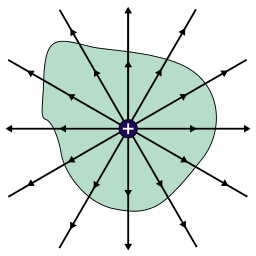Example of Gauss’s Law: A positive charge contained within a region of space creates an electric field that emanates from the surface of that region.

### Gauss’s Law for Magnetism

Gauss’s law for magnetism states that there are no “magnetic charges (or monopoles)” analogous to electric charges, and that magnetic fields are instead generated by magnetic dipoles. Such dipoles can be represented as loops of current, but in many ways are similar in appearance to positive and negative “magnetic charges” that are inseparable and thus have no formal net “magnetic charge.”

Magnetic field lines form loops such that all field lines that go into an object leave it at some point. Thus, the total magnetic flux through a surface surrounding a magnetic dipole is always zero.Field lines caused by a magnetic dipole: The field lines created by this magnetic dipole either form loops or extend infinitely.

The differential form of Gauss’s law for magnetic for magnetism is

$\nabla \cdot \bf \text{B}= 0$

Faraday’s law describes how a time-varying magnetic field (or flux) induces an electric field. The principle behind this phenomenon is used in many electric generators. Both macroscopic and microscopic differential equations are the same, relating electric field (E) to the time-partial derivative of magnetic field (B):

$\nabla \times \bf \text{E}=-\frac {\partial \bf \text{B}}{\partial \text{t}}$

### Ampere’s Circuital Law (with Maxwell’s correction)

Ampere’s law originally stated that magnetic field could be created by electrical current. Maxwell added a second source of magnetic fields in his correction: a changing electric field (or flux), which would induce a magnetic field even in the absence of an electrical current. He named the changing electric field “displacement current.”

Maxwell’s correction shows that self-sustaining electromagnetic waves (light) can travel through empty space even in the absence of moving charges or currents, with the electric field component and magnetic field component each continually changing and each perpetuating the other.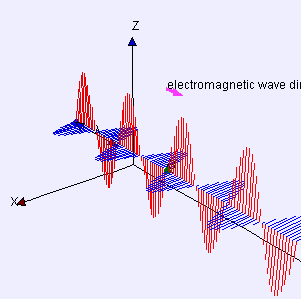Electromagnetic Waves: Electric (red) and magnetic (blue) waves propagate in phase sinusoidally, and perpendicularly to one another.

The microscopic approach to the Maxwell-corrected Ampere’s law relates magnetic field (B) to current density (J, or current per unit cross sectional area) and the time-partial derivative of electric field (E):

$\nabla \times \bf \text{B}=\mu_0 \bf \text{J} + \mu_0 \epsilon_0 \frac {\partial \bf \text{E}}{\partial \text{t}}$

## The Production of Electromagnetic Waves

Electromagnetic waves are the combination of electric and magnetic field waves produced by moving charges.

### Learning Objectives

Explain the self-perpetuating behavior of an electromagnetic wave

### Key Takeaways

#### Key Points

• Electromagnetic waves consist of both electric and magnetic field waves. These waves oscillate in perpendicular planes with respect to each other, and are in phase.
• The creation of all electromagnetic waves begins with an oscillating charged particle, which creates oscillating electric and magnetic fields.
• Once in motion, the electric and magnetic fields that a charged particle creates are self-perpetuating: time-dependent changes in one field (electric or magnetic) produce the other.

#### Key Terms

• electromagnetic wave: A wave of oscillating electric and magnetic fields.
• phase: Waves are said to be “in phase” when they begin at the same part (e.g., crest) of their respective cycles.

### Electromagnetic waves

Electromagnetic radiation, is a form of energy emitted by moving charged particles. As it travels through space it behaves like a wave, and has an oscillating electric field component and an oscillating magnetic field. These waves oscillate perpendicularly to and in phase with one another.Electromagnetic Wave: Electromagnetic waves are a self-propagating transverse wave of oscillating electric and magnetic fields. The direction of the electric field is indicated in blue, the magnetic field in red, and the wave propagates in the positive x-direction. Notice that the electric and magnetic field waves are in phase.

The creation of all electromagnetic waves begins with a charged particle. This charged particle creates an electric field (which can exert a force on other nearby charged particles). When it accelerates as part of an oscillatory motion, the charged particle creates ripples, or oscillations, in its electric field, and also produces a magnetic field (as predicted by Maxwell’s equations).

Once in motion, the electric and magnetic fields created by a charged particle are self-perpetuating—time-dependent changes in one field (electric or magnetic) produce the other. This means that an electric field that oscillates as a function of time will produce a magnetic field, and a magnetic field that changes as a function of time will produce an electric field. Both electric and magnetic fields in an electromagnetic wave will fluctuate in time, one causing the other to change.

Electromagnetic waves are ubiquitous in nature (i.e., light) and used in modern technology—AM and FM radio, cordless and cellular phones, garage door openers, wireless networks, radar, microwave ovens, etc. These and many more such devices use electromagnetic waves to transmit data and signals.

All the above sources of electromagnetic waves use the simple principle of moving charge, which can be easily modeled. Placing a coin in contact with both terminals of a 9-volt battery produces electromagnetic waves that can be detected by bringing the antenna of a radio (tuned to a static-producing station) within a few inches of the point of contact.

## Energy and Momentum

Electromagnetic waves have energy and momentum that are both associated with their wavelength and frequency.

### Learning Objectives

Relate energy of an electromagnetic wave with the frequency and wavelength

### Key Takeaways

#### Key Points

• Max Planck proved that energy of a photon (a stream of which is an electromagnetic wave ) is quantized and can exist in multiples of “Planck’s constant” (denoted as h, approximately equal to 6.626×10-34 J·s).
• $\text{E}=\text{hf}=\frac {\text{hc}}{\lambda}$ describes the energy (E) of a photon as a function of frequency (f), or wavelength (λ).
• $\text{p}=\frac {\text{E}}{\text{c}}=\frac {\text{hf}}{\text{c}}=\frac {\text{h}}{\lambda}$describes the momentum (p) of a photon as a function of its energy, frequency, or wavelength.

#### Key Terms

• photon: The quantum of light and other electromagnetic energy, regarded as a discrete particle having zero rest mass, no electric charge, and an indefinitely long lifetime.
• wavelength: The length of a single cycle of a wave, as measured by the distance between one peak or trough of a wave and the next; it is often designated in physics as λ, and corresponds to the velocity of the wave divided by its frequency.
• frequency: The quotient of the number of times n a periodic phenomenon occurs over the time t in which it occurs: f = n / t.

Electromagnetic radiation can essentially be described as photon streams. These photons are strictly defined as massless, but have both energy and surprisingly, given their lack of mass, momentum, which can be calculated from their wave properties.

Waves were poorly understood until the 1900s, when Max Planck and Albert Einstein developed modern corrections to classical theory.

Planck theorized that “black bodies” (thermal radiators) and other forms of electromagnetic radiation existed not as spectra, but in discrete, “quantized” form. In other words, there were only certain energies an electromagnetic wave could have. In his work he developed what is now known as “Planck’s constant,” which is approximately equal to 6.626×10-34 J·s.

### Energy

The energy (E) of a photon can be related to its frequency (f) by Planck’s constant (h):

$\text{E}=\text{hf}=\frac {\text{hc}}{\lambda}$

The ratio of speed of light (c) to wavelength (λ) can be substituted in place of f to give the same equation to energy in different terms. Note that energy cannot take any value: it can only exist in increments of frequency times Planck’s constant (or Planck’s constant times c divided by wavelength). Energy of a wave is therefore “quantized. ”Wavelength: Wavelength of the sinusoidal function is represented by λ.

### Momentum

Momentum is classically defined as the product of mass and velocity and thus would intuitively seem irrelevant to a discussion of electromagnetic radiation, which is both massless and composed of waves.

However, Einstein proved that light can act as particles in some circumstances, and that a wave-particle duality exists. And, given that he related energy and mass (E=mc2), it becomes more conceivable that a wave (which has an energy value) not only has an equation to mass but a momentum as well.

And indeed, Einstein proved that the momentum (p) of a photon is the ratio of its energy to the speed of light.

$\text{p}=\frac {\text{E}}{\text{c}}=\frac {\text{hf}}{\text{c}}=\frac {\text{h}}{\lambda}$

Substituting E with hc/λ cancels the c terms, making momentum also equal to the simple ratio of Planck’s constant to wavelength.

## The Speed of Light

The speed of light in a vacuum is one of the most fundamental constant in physics, playing a pivotal role in modern physics.

### Learning Objectives

Relate speed of light with the index of refraction of the medium

### Key Takeaways

#### Key Points

• The maximum possible value for the speed of light is that of light in a vacuum, and this speed is used for a constant in many area of physics.
• c is the symbol used to represent the speed of light in a vacuum, and its value is 299,792,458 meters per second.
• When light travels through medium, its speed is hindered by the index of refraction of that medium. Its actual speed can be found with: v=\frac{c}{n}.

#### Key Terms

• special relativity: A theory that (neglecting the effects of gravity) reconciles the principle of relativity with the observation that the speed of light is constant in all frames of reference.
• refractive index: The ratio of the speed of light in air or vacuum to that in another medium.

### The Speed of Light

The speed of light is generally a point of comparison to express that something is fast. shows a scale representation of the time it takes a beam of light to reach the moon from Earth. But what exactly is the speed of light?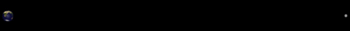Light Going from Earth to the Moon: A beam of light is depicted travelling between the Earth and the Moon in the time it takes a light pulse to move between them: 1.255 seconds at their mean orbital (surface-to-surface) distance. The relative sizes and separation of the Earth–Moon system are shown to scale.

It is just that: the speed of a photon or light particle. The speed of light in a vacuum (commonly written as c) is 299,792,458 meters per second. This is a universal physical constant used in many areas of physics. For example, you might be familiar with the equation:

$\text{E}=\text{mc}^2$,

where E = Energy and m = mass. This is known as the mass-energy equivalence, and it uses the speed of light to interrelate space and time. This not only explains the energy a body of mass contains, but also explains the hindrance mass has on speed.

There are many uses for the speed of light in a vacuum, such as in special relativity, which says that c is the natural speed limit and nothing can move faster than it. However, we know from our understanding of physics (and previous atoms) that the speed at which something travels also depends on the medium through which it is traveling. The speed at which light propagates through transparent materials (air, glass, etc.,) is dependent on the refractive index of that material, n:

$\text{v}=\frac{\text{c}}{\text{n}}$,

where v = actual velocity of light moving through the medium, c = speed of light in a vacuum, and n = refractive index of medium. The refractive index of air is about 1.0003, and from this equation we can find that the speed of visible light in air is about 90 km/s slower than c.

As mentioned earlier, the speed of light (usually of light in a vacuum) is used in many areas of physics. Below is an example of an application of the constant c.

### The Lorentz Factor

Fast-moving objects exhibit some properties that are counterintuitive from the perspective of classical mechanics. For example, length contracts and time dilates (runs slower) for objects in motion. The effects are typically minute, but are noticeable at sufficiently high speeds. The Lorentz factor (γ) is the factor by which length shortens and time dilates as a function of velocity (v):

$\gamma = (1-\text{v}^2/\text{c}^2)^{-1/2}$$\gamma = (1-\text{v}^2/\text{c}^2)^{-1/2}$$\gamma = (1-\text{v}^2/\text{c}^2)^{-1/2}$.

At low velocities, the quotient of v2/c2 is sufficiently close to 0 such that γ is approximately 1. However, as velocity approaches c, γ increases rapidly towards infinity.

## The Doppler Effect

The Doppler Effect is the change in a wave’s perceived frequency that results from the source’s motion, the observer, and the medium.

### Learning Objectives

Give examples of daily observations of the Doppler effect

### Key Takeaways

#### Key Points

• The Doppler effect is very commonly observed in action.
• The Doppler effect can be observed in the apparent change in pitch of a siren on an emergency vehicle, according to a stationary observer.
• The observer will notice the Doppler effect on the pitch of the stationary siren when moving relative to its pitch, or if the medium moves when the observer is stationary.

#### Key Terms

• doppler effect: Apparent change in frequency of a wave when the observer and the source of the wave move relative to each other.

### The Doppler Effect

The Doppler effect is a periodic event’s change in frequency for an observer in motion relative to the event’s source. Typically, this periodic event is a wave.

Most people have experienced the Doppler effect in action. Consider an emergency vehicle in motion, sounding its siren. As it approaches an observer, the pitch of the sound (its frequency) sounds higher than it actually is. When the vehicle reaches the observer, the pitch is perceived as it actually is. When the vehicle continues away from the observer, the pitch is perceived as lower than it actually is. From the perspective of an observer inside the vehicle, the pitch of the siren is constant.The Doppler Effect and Sirens: Waves emitted by a siren in a moving vehicle

The difference in the perceived pitch depending on observer location can be explained by the fact that the siren’s position changes as it emits waves. A wave of sound is emitted by a moving vehicle every millisecond. The vehicle ‘chases’ each wave in one direction. By the time the next wave is emitted, it is closer (relative to an onlooker ahead of the vehicle) to the previous wave than the wave’s frequency would suggest. Relative to an onlooker behind the vehicle, the second wave is further from the first wave than one would expect, which suggests a lower frequency.

The Doppler effect can be caused by any kind of motion. In the example above, the siren moved relative to a stationary observer. If the observer moves relative to the stationary siren, the observer will notice the Doppler effect on the pitch of the siren. Finally, if the medium through which the waves propagate moves, the Doppler effect will be noticed even for a stationary observer. An example of this phenomenon is wind.

Quantitatively, the Doppler effect can be characterized by relating the frequency perceived (f) to the velocity of waves in the medium (c), the velocity of the receiver relative to the medium (vr), the velocity of the source relative to the medium (vs), and the actual emitted frequency (f0):

$\text{f}=(\frac{\text{c}+\text{v}_\text{r}}{\text{c}+\text{v}_\text{s}})\text{f}_0$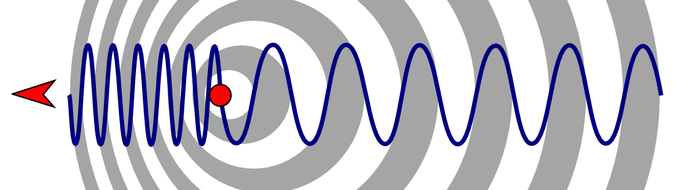The Doppler Effect: Wavelength change due to the motion of source

## Momentum Transfer and Radiation Pressure Atom

Radiation pressure is the pressure exerted upon any surface exposed to electromagnetic (EM) radiation.

### Key Takeaways

#### Key Points

• Photons carry momentum (p = E/c). When photons are absorbed or reflected on a surface, the surface receives momentum kicks. This momentum transfer leads to radiation pressure.
• Electromagnetic radiation applies radiation pressure equal to the Intensity (of light beam) divided by c (speed of light).
• Laser cooling uses radiation pressure to remove energy from atomic gases. The technique can produce cold samples of gases at 1mK or so.

#### Key Terms

• doppler effect: Apparent change in frequency of a wave when the observer and the source of the wave move relative to each other.
• classical electrodynamics: A branch of theoretical physics that studies consequences of the electromagnetic forces between electric charges and currents.

Radiation pressure is the pressure exerted upon any surface exposed to electromagnetic (EM) radiation. EM radiation (or photon, which is a quantum of light) carries momentum; this momentum is transferred to an object when the radiation is absorbed or reflected. Perhaps one of the most well know examples of the radiation pressure would be comet tails. Haley’s comet is shown in.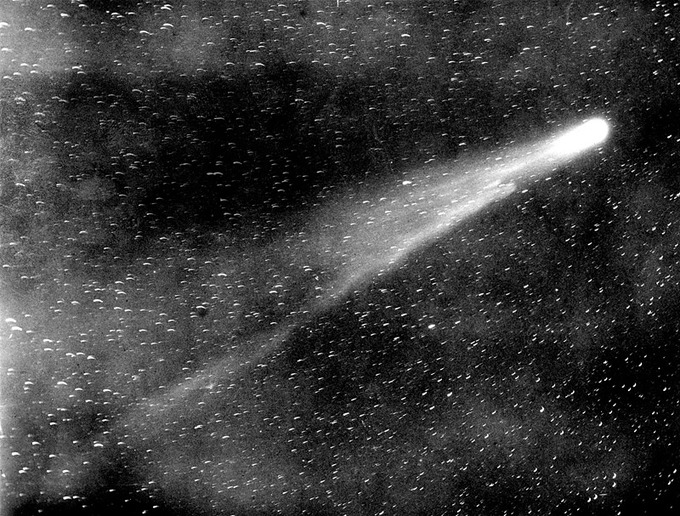Halley’s Comet: As a comet approaches the inner Solar System, solar radiation causes the volatile materials within the comet to vaporize and stream out of the nucleus. The streams of dust and gas thus released form an atmosphere around the comet (called the coma), and the force exerted on the coma by the Sun’s radiation pressure and solar wind cause the formation of an enormous tail that points away from the Sun.

Although radiation pressure can be understood using classical electrodynamics, here we will examine the quantum mechanical argument. From the perspective of quantum theory, light is made of photons: particles with zero mass but which carry energy and – importantly in this argument – momentum. According to special relativity, because photons are devoid of mass, their energy (E) and momentum (p) are related by E=pc.

Now consider a beam of light perpendicularly incident on a surface, and let us assume the beam of light is totally absorbed. The momentum the photons carry is a conserved quantity (i.e., it cannot be destroyed) so it must be transferred to the surface; thus the absorption of the light beam causes the surface to gain momentum. Newton’s Second Law tells us that force equals rate of change of momentum; thus during each second, the surface experiences a force (or pressure, as pressure is force per unit area) due to the momentum the photons transfer to it.

This gives us: pressure = momentum transferred per second per unit area = energy deposited per second per unit area / c = I/c, (where I is the intensity of the beam of light).

### Laser Cooling

There are many variations of laser cooling, but they all use radiation pressure to remove energy from atomic gases (and therefore cool the sample). In laser cooling (sometimes called Doppler cooling), the frequency of light is tuned slightly below an electronic transition in the atom. Because light is detuned to the “red” (i.e., at lower frequency) of the transition, the atoms will absorb more photons if they move towards the light source, due to the Doppler effect. Thus if one applies light from two opposite directions, the atoms will always scatter more photons from the laser beam pointing opposite to their direction of motion (typical setups applies three opposing pairs of laser beams as in ).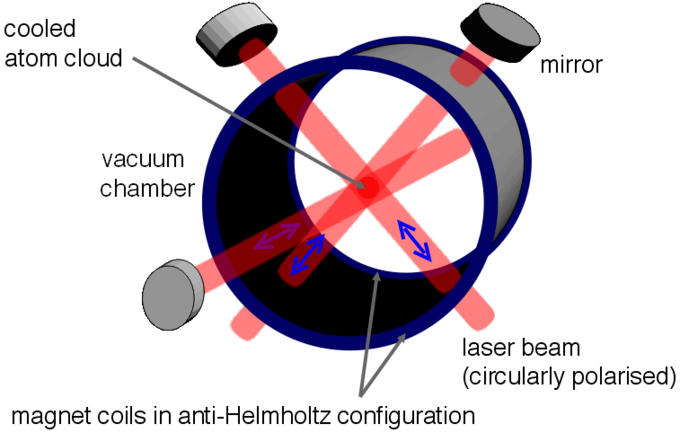The Magneto Optical Trap: Experimental setup of Magneto Optical Trap (MOT), which uses radiation pressure to cool atomic species. Atoms are slowed down by absorbing (and emitting) photons.

In each scattering event, the atom loses a momentum equal to the momentum of the photon. If the atom (which is now in the excited state) then emits a photon spontaneously, it will be kicked by the same amount of momentum, only in a random direction. Since the initial momentum loss was opposite to the direction of motion (while the subsequent momentum gain was in a random direction), the overall result of the absorption and emission process is to reduce the speed of the atom. If the absorption and emission are repeated many times, the average speed (and therefore the kinetic energy ) of the atom will be reduced. Since the temperature of a group of atoms is a measure of the average random internal kinetic energy, this is equivalent to cooling the atoms. Simple laser cooling setups can produce a cold sample of atomic gases at around 1mK (=10-3 K) starting from a room temperature gas.